# FOURIER SERIESEvery periodic function f(t) which has the period T 2Tt/oand has certain continuity conditions can be represented by aseries plus a constantfAt)= aa, cos (noof)b,sin (n)п %3The above holds iff(t) has a continuous derivative f'(t) forall t. It should be noted that the various sinusoids present inthe series are orthogonal on the interval 0 to T and as a resultthe coefficients are given by0(1/T)t)dta, (2/T)t) cos (nf)dt(2/T)t)sin(nwof)dt-T1,2, ...п %3DCOS1,2,..П —The constants anand bn are the Fourier coefficients of f(t) forthe interval 0 to T and the corresponding series is called theFourier series of f(t) over the same interval. SRasdeAo=3 Cosnwt ctsinsnwnusSinwsSinnwatdlACOSShws1)5nwsPEL= 3 +S6SintnussCoSsnw t6COS(SnWa 65nwoSin pwot

Question
30 views

Hi,

kindly advise if my answer is accurate or not based on the given Fourier series coefficients.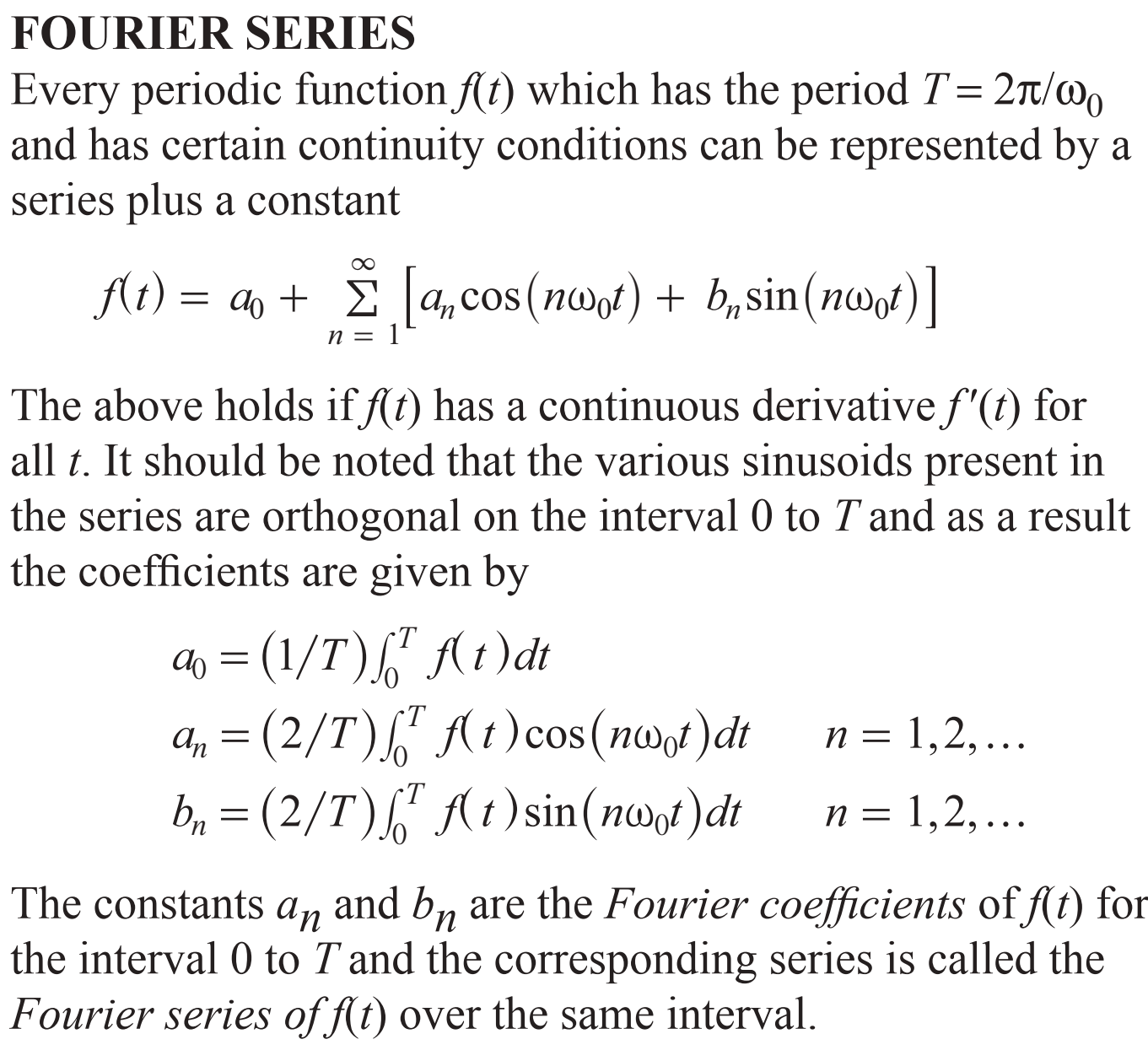help_outlineImage TranscriptioncloseFOURIER SERIES Every periodic function f(t) which has the period T 2Tt/o and has certain continuity conditions can be represented by a series plus a constant fAt)= a a, cos (noof)b,sin (n) п %3 The above holds iff(t) has a continuous derivative f'(t) for all t. It should be noted that the various sinusoids present in the series are orthogonal on the interval 0 to T and as a result the coefficients are given by 0(1/T)t)dt a, (2/T)t) cos (nf)dt (2/T)t)sin(nwof)dt -T 1,2, ... п %3D COS 1,2,.. П — The constants an and bn are the Fourier coefficients of f(t) for the interval 0 to T and the corresponding series is called the Fourier series of f(t) over the same interval. fullscreen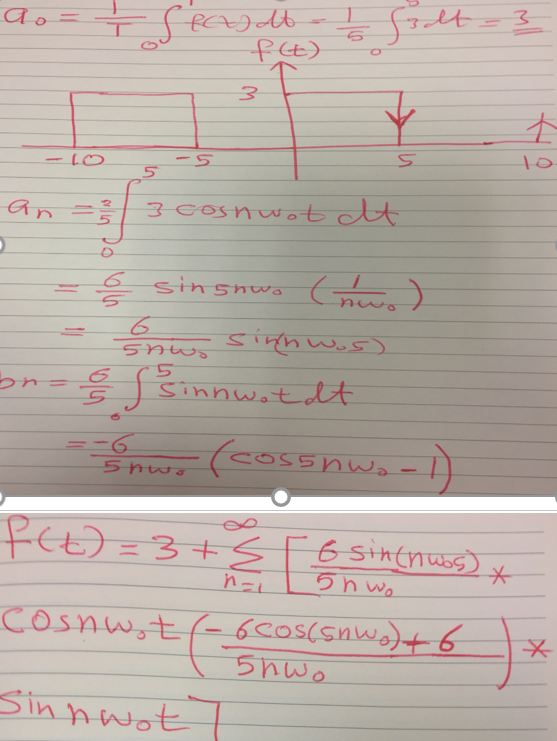help_outlineImage TranscriptioncloseSRasde Ao= 3 Cosnwt ct sinsnw nus Sinws SinnwatdlA COSShws1) 5nws PEL= 3 +S 6Sintnuss CoSsnw t 6COS(SnWa 6 5nwo Sin pwot fullscreen
check_circle

star
star
star
star
star
1 Rating
Step 1

The solution for the given Fourier series problem can be obtained as follows. Compute the coefficient a0 as follows.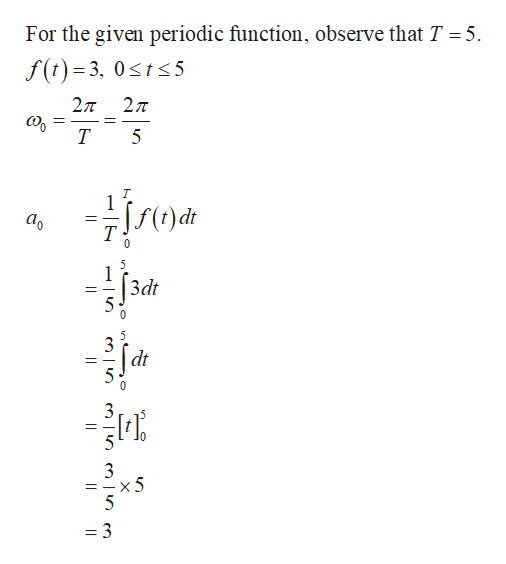help_outlineImage TranscriptioncloseFor the given periodic function, observe that T =5 f(t)= 3, 0sts 5 27T 27T T 5 T 1 аo 5 3dt 5 0 3 dt x 5 5 = 3 X fullscreen
Step 2

Compute the coefficient an as follows.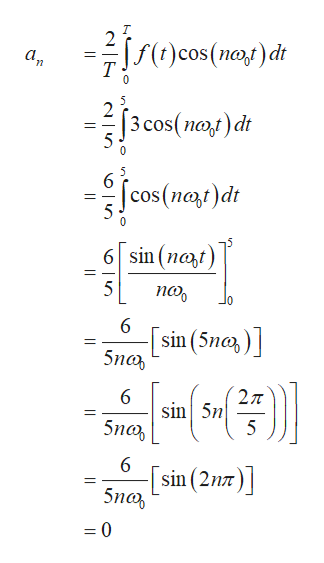help_outlineImage Transcriptionclose)cos()d 2 а, 0 5 2 |3 cos(пo,f) dr 6 |cos(nat)dt 11 6 sin (nat 5 по, 6 sin (5na) 5nan 2T sin 5n 5 5na 6 sin (2n 5no =0 fullscreen
Step 3

Compute the coefficient bn as follows.   ...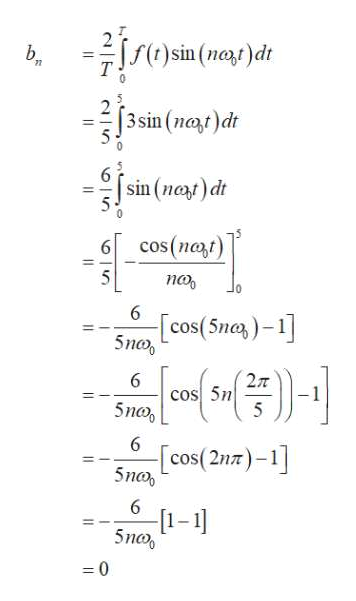help_outlineImage Transcriptionclosesin(n)dr b 11 2 [3 sin (net)dt 6 sin (na)dt cos (nert) 6 5 по, 6 Sncos(5na)-1 6 cos 5n 5no 5 6 [cos(2n7)-1 5no, 6 -[1-1 5nco 0 II fullscreen

### Want to see the full answer?

See Solution

#### Want to see this answer and more?

Solutions are written by subject experts who are available 24/7. Questions are typically answered within 1 hour.*

See Solution
*Response times may vary by subject and question.
Tagged in

### Calculus